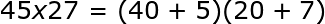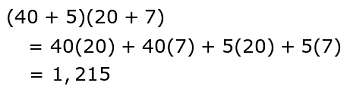# How to Do Double Digit Multiplication: Steps & Practice Problems

An error occurred trying to load this video.

Try refreshing the page, or contact customer support.

Coming up next: How to Teach Double Digit Multiplication

### You're on a roll. Keep up the good work!

Replay
Your next lesson will play in 10 seconds
• 0:02 Multiplication Methods
• 0:40 Box Method
• 2:02 Distribution Method
• 2:51 Column Method
• 3:45 Lesson Summary

Want to watch this again later?

Timeline
Autoplay
Autoplay
Speed

#### Recommended Courses for You

Lesson Transcript
Instructor: Mia Primas

Mia has taught math and science and has a Master's Degree in Secondary Teaching.

In this video, we'll explore different strategies for multiplying numbers with two digits, including the box, distribution and column methods, and use examples to see how they work.

## Multiplication Methods

Teachers often have the challenge of instructing students that have different learning styles and abilities. For this reason, they need to offer them more than one strategy for solving a problem. Some students prefer a systematic process, while others prefer to use visual aids. It is also important for students to understand why a process works, rather than simply to follow the steps that a teacher shows them. In this lesson, we will discuss three methods for double-digit multiplication. They are the box method, distribution method, and column method; after which you can decide which one you prefer to use.

## Box Method

While the column method is the most commonly used strategy for multiplication, the box method is useful for understanding why the column method works. For this reason, we will look at the box method first and illustrate it with an example.

#### Example 1

Find the product of 34 and 12.

The first step in the box method is to write each number in an expanded form, which is a way of writing numbers that shows the value of its digits. For example, in the number 34, the three is in the tens place so it is written as three tens, or 30. The four is written in the ones place so it has a value of four ones, or 4. In expanded form, we show 34 as 30 + 4 and 12 is 10 + 2.

Once we've written a number in expanded form, we can enter them into the table. To fill in the remaining spaces in the table, we multiply the numbers that represent the row and column for each box.

 30 4 10 300 40 2 60 8

The final step is to add the numbers in the boxes together, giving us 408.

300 + 40 + 60 + 8 = 408

Therefore, product of 34 and 12 is 408.

## Distribution Method

Let's look at another example while we are discussing the distribution method.

#### Example 2

Find the product of 45 and 27.

The distribution method uses a similar strategy as the box method, but without having to draw the boxes. Instead the numbers are written in expanded form, and then placed in parentheses.Next the distributive property is used to multiply the numbers. The distributive property tells us to multiply each number in the first set of parentheses by each number in the second set of parentheses. Lastly, we add the numbers together to get the product.To unlock this lesson you must be a Study.com Member.

### Register to view this lesson

Are you a student or a teacher?

### Unlock Your Education

#### See for yourself why 30 million people use Study.com

##### Become a Study.com member and start learning now.
Back
What teachers are saying about Study.com

### Earning College Credit

Did you know… We have over 160 college courses that prepare you to earn credit by exam that is accepted by over 1,500 colleges and universities. You can test out of the first two years of college and save thousands off your degree. Anyone can earn credit-by-exam regardless of age or education level.